• ## 教你彻底学会动态规划——入门篇

万次阅读 多人点赞 2015-08-11 13:26:41
动态规划相信大家都知道，动态规划算法也是新手在刚接触算法设计时很苦恼的问题，有时候觉得难以理解，但是真正理解之后，就会觉得动态规划其实并没有想象中那么难。网上也有很多关于讲解动态规划的文章，大多都是...
    动态规划相信大家都知道，动态规划算法也是新手在刚接触算法设计时很苦恼的问题，有时候觉得难以理解，但是真正理解之后，就会觉得动态规划其实并没有想象中那么难。网上也有很多关于讲解动态规划的文章，大多都是叙述概念，讲解原理，让人觉得晦涩难懂，即使一时间看懂了，发现当自己做题的时候又会觉得无所适从。我觉得，理解算法最重要的还是在于练习，只有通过自己练习，才可以更快地提升。话不多说，接下来，下面我就通过一个例子来一步一步讲解动态规划是怎样使用的，只有知道怎样使用，才能更好地理解，而不是一味地对概念和原理进行反复琢磨。

首先，我们看一下这道题（此题目来源于北大POJ）：

数字三角形(POJ1163)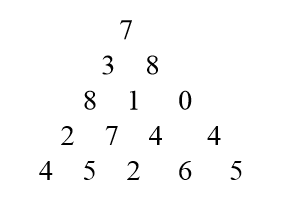在上面的数字三角形中寻找一条从顶部到底边的路径，使得路径上所经过的数字之和最大。路径上的每一步都只能往左下或 右下走。只需要求出这个最大和即可，不必给出具体路径。 三角形的行数大于1小于等于100，数字为 0 - 99

输入格式：

5      //表示三角形的行数    接下来输入三角形

7

3   8

8   1   0

2   7   4   4

4   5   2   6   5

要求输出最大和

接下来，我们来分析一下解题思路：

首先，肯定得用二维数组来存放数字三角形

然后我们用D( r, j) 来表示第r行第 j 个数字(r,j从1开始算)

我们用MaxSum(r, j)表示从D(r,j)到底边的各条路径中，最佳路径的数字之和。

因此，此题的最终问题就变成了求 MaxSum(1,1)

当我们看到这个题目的时候，首先想到的就是可以用简单的递归来解题：

D(r, j)出发，下一步只能走D(r+1,j)或者D(r+1, j+1)。故对于N行的三角形，我们可以写出如下的递归式：

if ( r == N)
MaxSum(r,j) = D(r,j)
else
MaxSum( r, j) = Max{ MaxSum(r＋1,j), MaxSum(r+1,j+1) } + D(r,j) 

根据上面这个简单的递归式，我们就可以很轻松地写出完整的递归代码：

#include <iostream>
#include <algorithm>
#define MAX 101
using namespace std;
int D[MAX][MAX];
int n;
int MaxSum(int i, int j){
if(i==n)
return D[i][j];
int x = MaxSum(i+1,j);
int y = MaxSum(i+1,j+1);
return max(x,y)+D[i][j];
}
int main(){
int i,j;
cin >> n;
for(i=1;i<=n;i++)
for(j=1;j<=i;j++)
cin >> D[i][j];
cout << MaxSum(1,1) << endl;
}      

对于如上这段递归的代码，当我提交到POJ时，会显示如下结果：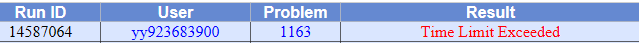对的，代码运行超时了，为什么会超时呢？

答案很简单，因为我们重复计算了，当我们在进行递归时，计算机帮我们计算的过程如下图：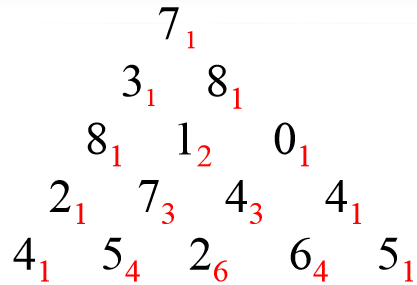就拿第三行数字1来说，当我们计算从第2行的数字3开始的MaxSum时会计算出从1开始的MaxSum，当我们计算从第二行的数字8开始的MaxSum的时候又会计算一次从1开始的MaxSum，也就是说有重复计算。这样就浪费了大量的时间。也就是说如果采用递规的方法，深度遍历每条路径，存在大量重复计算。则时间复杂度为 2的n次方,对于 n = 100 行，肯定超时。

接下来，我们就要考虑如何进行改进，我们自然而然就可以想到如果每算出一个MaxSum(r,j)就保存起来，下次用到其值的时候直接取用，则可免去重复计算。那么可以用n方的时间复杂度完成计算。因为三角形的数字总数是 n(n+1)/2

根据这个思路，我们就可以将上面的代码进行改进，使之成为记忆递归型的动态规划程序：

#include <iostream>
#include <algorithm>
using namespace std;

#define MAX 101

int D[MAX][MAX];
int n;
int maxSum[MAX][MAX];

int MaxSum(int i, int j){
if( maxSum[i][j] != -1 )
return maxSum[i][j];
if(i==n)
maxSum[i][j] = D[i][j];
else{
int x = MaxSum(i+1,j);
int y = MaxSum(i+1,j+1);
maxSum[i][j] = max(x,y)+ D[i][j];
}
return maxSum[i][j];
}
int main(){
int i,j;
cin >> n;
for(i=1;i<=n;i++)
for(j=1;j<=i;j++) {
cin >> D[i][j];
maxSum[i][j] = -1;
}
cout << MaxSum(1,1) << endl;
} 

当我们提交如上代码时，结果就是一次AC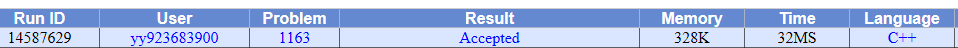虽然在短时间内就AC了。但是，我们并不能满足于这样的代码，因为递归总是需要使用大量堆栈上的空间，很容易造成栈溢出，我们现在就要考虑如何把递归转换为递推，让我们一步一步来完成这个过程。

我们首先需要计算的是最后一行，因此可以把最后一行直接写出，如下图：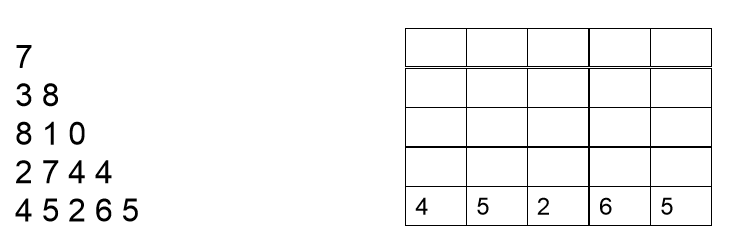现在开始分析倒数第二行的每一个数，现分析数字2，2可以和最后一行4相加，也可以和最后一行的5相加，但是很显然和5相加要更大一点，结果为7，我们此时就可以将7保存起来，然后分析数字7，7可以和最后一行的5相加，也可以和最后一行的2相加，很显然和5相加更大，结果为12，因此我们将12保存起来。以此类推。。我们可以得到下面这张图：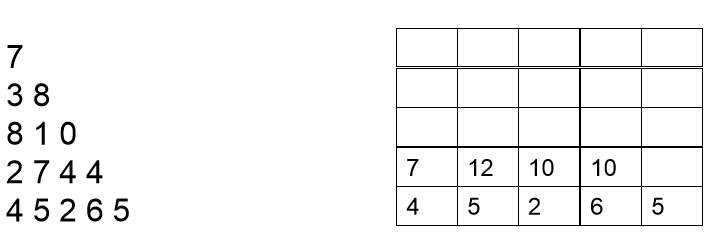然后按同样的道理分析倒数第三行和倒数第四行，最后分析第一行，我们可以依次得到如下结果：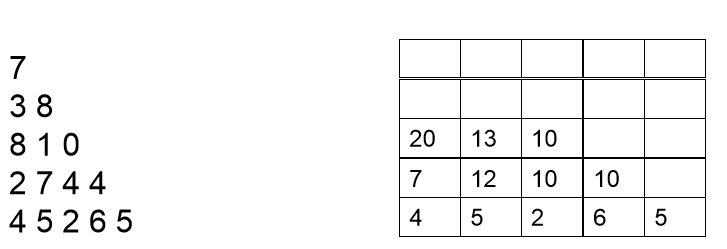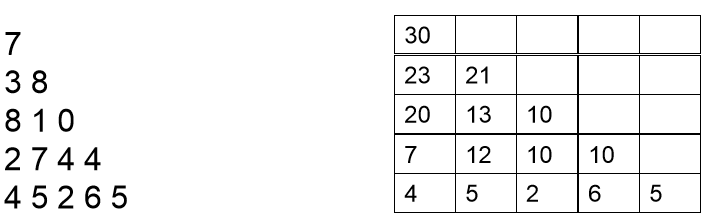上面的推导过程相信大家不难理解，理解之后我们就可以写出如下的递推型动态规划程序：

#include <iostream>
#include <algorithm>
using namespace std;

#define MAX 101

int D[MAX][MAX];
int n;
int maxSum[MAX][MAX];
int main(){
int i,j;
cin >> n;
for(i=1;i<=n;i++)
for(j=1;j<=i;j++)
cin >> D[i][j];
for( int i = 1;i <= n; ++ i )
maxSum[n][i] = D[n][i];
for( int i = n-1; i>= 1;  --i )
for( int j = 1; j <= i; ++j )
maxSum[i][j] = max(maxSum[i+1][j],maxSum[i+1][j+1]) + D[i][j];
cout << maxSum << endl;
} 

我们的代码仅仅是这样就够了吗？当然不是，我们仍然可以继续优化，而这个优化当然是对于空间进行优化，其实完全没必要用二维maxSum数组存储每一个MaxSum(r,j),只要从底层一行行向上递推，那么只要一维数组maxSum即可,即只要存储一行的MaxSum值就可以。

对于空间优化后的具体递推过程如下：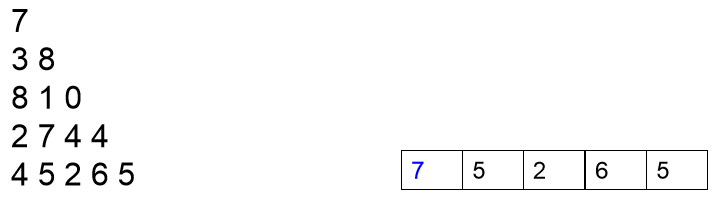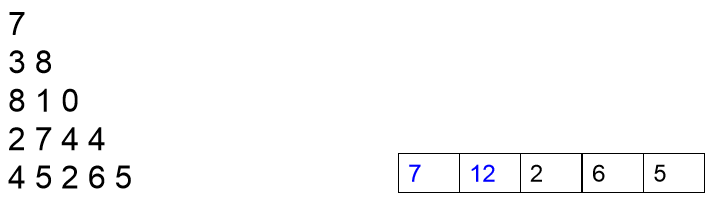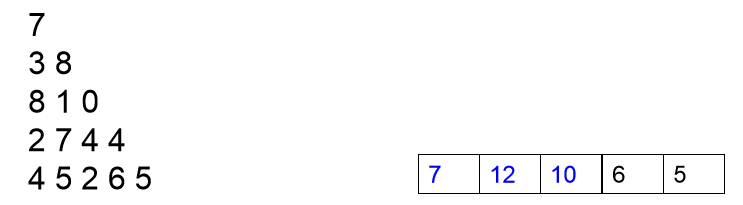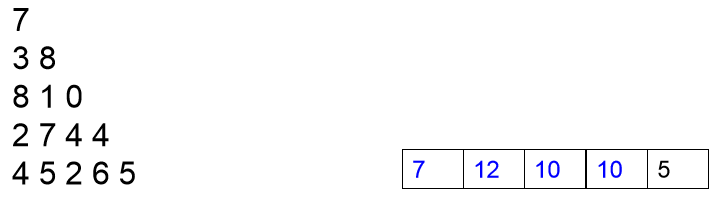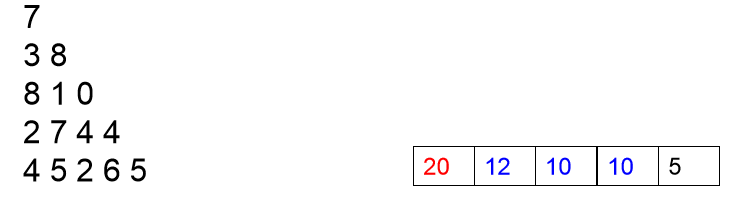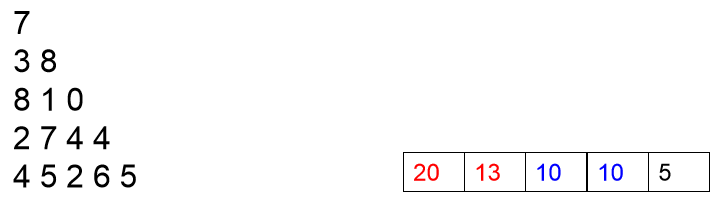接下里的步骤就按上图的过程一步一步推导就可以了。进一步考虑，我们甚至可以连maxSum数组都可以不要，直接用D的第n行直接替代maxSum即可。但是这里需要强调的是：虽然节省空间，但是时间复杂度还是不变的。

依照上面的方式，我们可以写出如下代码：

#include <iostream>
#include <algorithm>
using namespace std;

#define MAX 101

int D[MAX][MAX];
int n;
int * maxSum;

int main(){
int i,j;
cin >> n;
for(i=1;i<=n;i++)
for(j=1;j<=i;j++)
cin >> D[i][j];
maxSum = D[n]; //maxSum指向第n行
for( int i = n-1; i>= 1;  --i )
for( int j = 1; j <= i; ++j )
maxSum[j] = max(maxSum[j],maxSum[j+1]) + D[i][j];
cout << maxSum << endl;
}

接下来，我们就进行一下总结：

递归到动规的一般转化方法

递归函数有n个参数，就定义一个n维的数组，数组的下标是递归函数参数的取值范围，数组元素的值是递归函数的返回值，这样就可以从边界值开始， 逐步填充数组，相当于计算递归函数值的逆过程。

动规解题的一般思路

1. 将原问题分解为子问题

把原问题分解为若干个子问题，子问题和原问题形式相同或类似，只不过规模变小了。子问题都解决，原问题即解决(数字三角形例）。
子问题的解一旦求出就会被保存，所以每个子问题只需求 解一次。
2.确定状态

在用动态规划解题时，我们往往将和子问题相关的各个变量的一组取值，称之为一个“状 态”。一个“状态”对应于一个或多个子问题， 所谓某个“状态”下的“值”，就是这个“状 态”所对应的子问题的解。
所有“状态”的集合，构成问题的“状态空间”。“状态空间”的大小，与用动态规划解决问题的时间复杂度直接相关。 在数字三角形的例子里，一共有N×(N+1)/2个数字，所以这个问题的状态空间里一共就有N×(N+1)/2个状态。
整个问题的时间复杂度是状态数目乘以计算每个状态所需时间。在数字三角形里每个“状态”只需要经过一次，且在每个状态上作计算所花的时间都是和N无关的常数。

3.确定一些初始状态（边界状态）的值

以“数字三角形”为例，初始状态就是底边数字，值就是底边数字值。

4. 确定状态转移方程

定义出什么是“状态”，以及在该“状态”下的“值”后，就要找出不同的状态之间如何迁移――即如何从一个或多个“值”已知的 “状态”，求出另一个“状态”的“值”(递推型)。状态的迁移可以用递推公式表示，此递推公式也可被称作“状态转移方程”。

数字三角形的状态转移方程: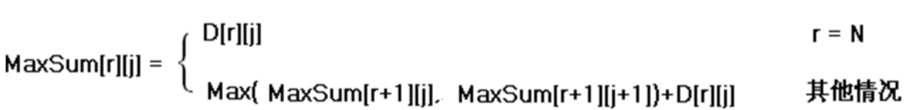能用动规解决的问题的特点

1) 问题具有最优子结构性质。如果问题的最优解所包含的 子问题的解也是最优的，我们就称该问题具有最优子结 构性质。

2) 无后效性。当前的若干个状态值一旦确定，则此后过程的演变就只和这若干个状态的值有关，和之前是采取哪种手段或经过哪条路径演变到当前的这若干个状态，没有关系。

好久没看博客发现这篇文章现在已经这么火热了，看了一下评论发现不少人对这篇文章都比较有兴趣，我当初写这篇文章是受到了Coursera上面一门算法课程的启发，大家有兴趣可以去听听这门课程：数据结构与算法


展开全文c 算法
• 动态规划难吗？说实话，我觉得很难，特别是对于初学者来说，我当时入门动态规划的时候，是看 0-1 背包问题，当时真的是一脸懵逼。后来，我遇到动态规划的题，看的懂答案，但就是自己不会做，不知道怎么下手。就像做...
动态规划难吗？说实话，我觉得很难，特别是对于初学者来说，我当时入门动态规划的时候，是看 0-1 背包问题，当时真的是一脸懵逼。后来，我遇到动态规划的题，看的懂答案，但就是自己不会做，不知道怎么下手。就像做递归的题，看的懂答案，但下不了手，关于递归的，我之前也写过一篇套路的文章，如果对递归不大懂的，强烈建议看一看：为什么你学不会递归，告别递归，谈谈我的经验
对于动态规划，春招秋招时好多题都会用到动态规划，一气之下，再 leetcode 连续刷了几十道题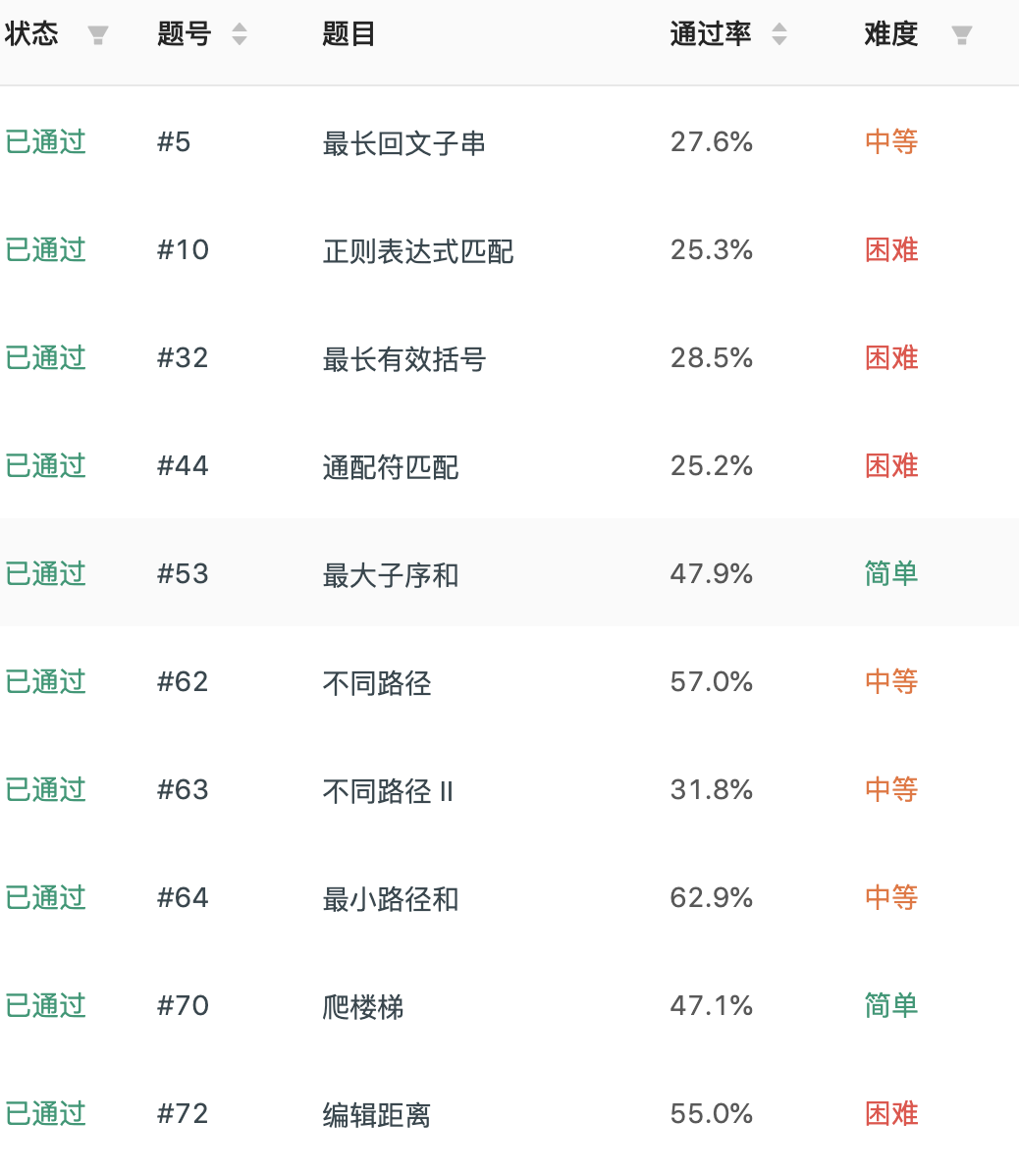之后，豁然开朗 ，感觉动态规划也不是很难，今天，我就来跟大家讲一讲，我是怎么做动态规划的题的，以及从中学到的一些套路。相信你看完一定有所收获
如果你对动态规划感兴趣，或者你看的懂动态规划，但却不知道怎么下手，那么我建议你好好看以下，这篇文章的写法，和之前那篇讲递归的写法，是差不多一样的，将会举大量的例子。如果一次性看不完，建议收藏，同时别忘了素质三连。

为了兼顾初学者，我会从最简单的题讲起，后面会越来越难，最后面还会讲解，该如何优化。因为 80% 的动规都是可以进行优化的。不过我得说，如果你连动态规划是什么都没听过，可能这篇文章你也会压力山大。

一、动态规划的三大步骤
动态规划，无非就是利用历史记录，来避免我们的重复计算。而这些历史记录，我们得需要一些变量来保存，一般是用一维数组或者二维数组来保存。下面我们先来讲下做动态规划题很重要的三个步骤，

如果你听不懂，也没关系，下面会有很多例题讲解，估计你就懂了。之所以不配合例题来讲这些步骤，也是为了怕你们脑袋乱了

第一步骤：定义数组元素的含义，上面说了，我们会用一个数组，来保存历史数组，假设用一维数组 dp[] 吧。这个时候有一个非常非常重要的点，就是规定你这个数组元素的含义，例如你的 dp[i] 是代表什么意思？
第二步骤：找出数组元素之间的关系式，我觉得动态规划，还是有一点类似于我们高中学习时的归纳法的，当我们要计算 dp[n] 时，是可以利用 dp[n-1]，dp[n-2]…dp，来推出 dp[n] 的，也就是可以利用历史数据来推出新的元素值，所以我们要找出数组元素之间的关系式，例如 dp[n] = dp[n-1] + dp[n-2]，这个就是他们的关系式了。而这一步，也是最难的一步，后面我会讲几种类型的题来说。

学过动态规划的可能都经常听到最优子结构，把大的问题拆分成小的问题，说时候，最开始的时候，我是对最优子结构一梦懵逼的。估计你们也听多了，所以这一次，我将换一种形式来讲，不再是各种子问题，各种最优子结构。所以大佬可别喷我再乱讲，因为我说了，这是我自己平时做题的套路。

第三步骤：找出初始值。学过数学归纳法的都知道，虽然我们知道了数组元素之间的关系式，例如 dp[n] = dp[n-1] + dp[n-2]，我们可以通过 dp[n-1] 和 dp[n-2] 来计算 dp[n]，但是，我们得知道初始值啊，例如一直推下去的话，会由 dp = dp + dp。而 dp 和 dp 是不能再分解的了，所以我们必须要能够直接获得 dp 和 dp 的值，而这，就是所谓的初始值。
由了初始值，并且有了数组元素之间的关系式，那么我们就可以得到 dp[n] 的值了，而 dp[n] 的含义是由你来定义的，你想求什么，就定义它是什么，这样，这道题也就解出来了。
不懂？没事，我们来看三四道例题，我讲严格按这个步骤来给大家讲解。
二、案例详解
案例一、简单的一维 DP

问题描述：一只青蛙一次可以跳上1级台阶，也可以跳上2级。求该青蛙跳上一个n级的台阶总共有多少种跳法。

(1)、定义数组元素的含义
按我上面的步骤说的，首先我们来定义 dp[i] 的含义，我们的问题是要求青蛙跳上 n 级的台阶总共由多少种跳法，那我们就定义 dp[i] 的含义为：跳上一个 i 级的台阶总共有 dp[i] 种跳法。这样，如果我们能够算出 dp[n]，不就是我们要求的答案吗？所以第一步定义完成。
（2）、找出数组元素间的关系式
我们的目的是要求 dp[n]，动态规划的题，如你们经常听说的那样，就是把一个规模比较大的问题分成几个规模比较小的问题，然后由小的问题推导出大的问题。也就是说，dp[n] 的规模为 n，比它规模小的是 n-1, n-2, n-3… 也就是说，dp[n] 一定会和 dp[n-1], dp[n-2]…存在某种关系的。我们要找出他们的关系。
那么问题来了，怎么找？
这个怎么找，是最核心最难的一个，我们必须回到问题本身来了，来寻找他们的关系式，dp[n] 究竟会等于什么呢？
对于这道题，由于情况可以选择跳一级，也可以选择跳两级，所以青蛙到达第 n 级的台阶有两种方式
一种是从第 n-1 级跳上来
一种是从第 n-2 级跳上来
由于我们是要算所有可能的跳法的，所以有 dp[n] = dp[n-1] + dp[n-2]。
（3）、找出初始条件
当 n = 1 时，dp = dp + dp[-1]，而我们是数组是不允许下标为负数的，所以对于 dp，我们必须要直接给出它的数值，相当于初始值，显然，dp = 1。一样，dp = 0.（因为 0 个台阶，那肯定是 0 种跳法了）。于是得出初始值：
dp = 0.
dp = 1.
即 n <= 1 时，dp[n] = n.
三个步骤都做出来了，那么我们就来写代码吧，代码会详细注释滴。
int f( int n ){
if(n <= 1)
return n;
// 先创建一个数组来保存历史数据
int[] dp = new int[n+1];
// 给出初始值
dp = 0;
dp = 1;
// 通过关系式来计算出 dp[n]
for(int i = 2; i <= n; i++){
dp[i] = dp[i-1] + dp[-2];
}
// 把最终结果返回
return dp[n];
}

（4）、再说初始化
大家先想以下，你觉得，上面的代码有没有问题？
答是有问题的，还是错的，错在对初始值的寻找不够严谨，这也是我故意这样弄的，意在告诉你们，关于初始值的严谨性。例如对于上面的题，当 n = 2 时，dp = dp + dp = 1。这显然是错误的，你可以模拟一下，应该是 dp = 2。
也就是说，在寻找初始值的时候，一定要注意不要找漏了，dp 也算是一个初始值，不能通过公式计算得出。有人可能会说，我想不到怎么办？这个很好办，多做几道题就可以了。

下面我再列举三道不同的例题，并且，再在未来的文章中，我也会持续按照这个步骤，给大家找几道有难度且类型不同的题。下面这几道例题，不会讲的特性详细哈。实际上 ，上面的一维数组是可以把空间优化成更小的，不过我们现在先不讲优化的事，下面的题也是，不讲优化版本。

案例二：二维数组的 DP
我做了几十道 DP 的算法题，可以说，80% 的题，都是要用二维数组的，所以下面的题主要以二维数组为主，当然有人可能会说，要用一维还是二维，我怎么知道？这个问题不大，接着往下看。
问题描述
一个机器人位于一个 m x n 网格的左上角 （起始点在下图中标记为“Start” ）。
机器人每次只能向下或者向右移动一步。机器人试图达到网格的右下角（在下图中标记为“Finish”）。
问总共有多少条不同的路径？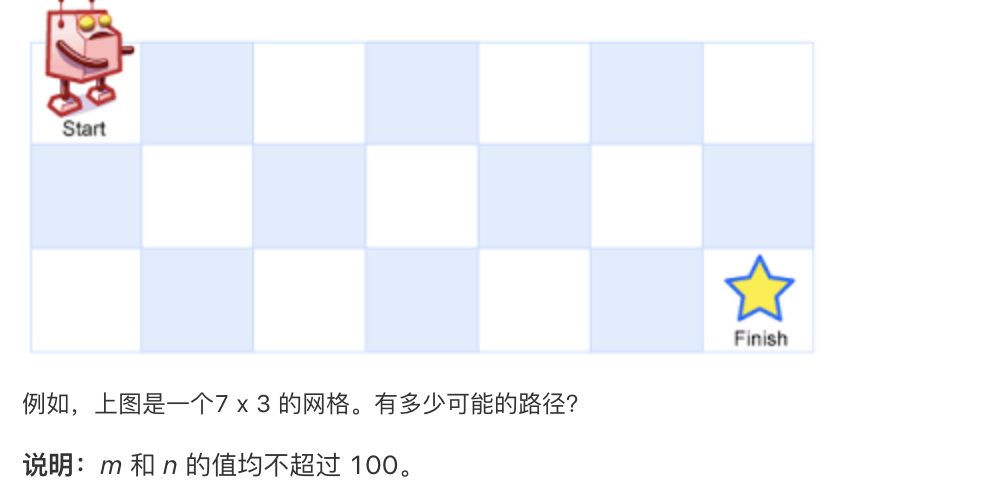这是 leetcode 的 62 号题：https://leetcode-cn.com/problems/unique-paths/

还是老样子，三个步骤来解决。
步骤一、定义数组元素的含义
由于我们的目的是从左上角到右下角一共有多少种路径，那我们就定义 dp[i] [j]的含义为：当机器人从左上角走到(i, j) 这个位置时，一共有 dp[i] [j] 种路径。那么，dp[m-1] [n-1] 就是我们要的答案了。

注意，这个网格相当于一个二维数组，数组是从下标为 0 开始算起的，所以 右下角的位置是 (m-1, n - 1)，所以 dp[m-1] [n-1] 就是我们要找的答案。

步骤二：找出关系数组元素间的关系式
想象以下，机器人要怎么样才能到达 (i, j) 这个位置？由于机器人可以向下走或者向右走，所以有两种方式到达
一种是从 (i-1, j) 这个位置走一步到达
一种是从(i, j - 1) 这个位置走一步到达
因为是计算所有可能的步骤，所以是把所有可能走的路径都加起来，所以关系式是 dp[i] [j] = dp[i-1] [j] + dp[i] [j-1]。
步骤三、找出初始值
显然，当 dp[i] [j] 中，如果 i 或者 j 有一个为 0，那么还能使用关系式吗？答是不能的，因为这个时候把 i - 1 或者 j - 1，就变成负数了，数组就会出问题了，所以我们的初始值是计算出所有的 dp [0….n-1] 和所有的 dp[0….m-1] 。这个还是非常容易计算的，相当于计算机图中的最上面一行和左边一列。因此初始值如下：
dp [0….n-1] = 1; // 相当于最上面一行，机器人只能一直往左走
dp[0…m-1]  = 1; // 相当于最左面一列，机器人只能一直往下走
撸代码
三个步骤都写出来了，直接看代码
public static int uniquePaths(int m, int n) {
if (m <= 0 || n <= 0) {
return 0;
}

int[][] dp = new int[m][n]; //
// 初始化
for(int i = 0; i < m; i++){
dp[i] = 1;
}
for(int i = 0; i < n; i++){
dp[i] = 1;
}
// 推导出 dp[m-1][n-1]
for (int i = 1; i < m; i++) {
for (int j = 1; j < n; j++) {
dp[i][j] = dp[i-1][j] + dp[i][j-1];
}
}
return dp[m-1][n-1];
}


O(n*m) 的空间复杂度可以优化成 O(min(n, m)) 的空间复杂度的，不过这里先不讲

案例三、二维数组 DP
写到这里，有点累了，，但还是得写下去，所以看的小伙伴，你们可得继续看呀。下面这道题也不难，比上面的难一丢丢，不过也是非常类似
问题描述
给定一个包含非负整数的 m x n 网格，请找出一条从左上角到右下角的路径，使得路径上的数字总和为最小。
**说明：**每次只能向下或者向右移动一步。
举例：
输入:
arr = [
[1,3,1],
[1,5,1],
[4,2,1]
]
输出: 7
解释: 因为路径 1→3→1→1→1 的总和最小。

和上面的差不多，不过是算最优路径和，这是 leetcode 的第64题：https://leetcode-cn.com/problems/minimum-path-sum/

还是老样子，可能有些人都看烦了，哈哈，但我还是要按照步骤来写，让那些不大懂的加深理解。有人可能觉得，这些题太简单了吧，别慌，小白先入门，这些属于 medium 级别的，后面在给几道 hard 级别的。

步骤一、定义数组元素的含义
由于我们的目的是从左上角到右下角，最小路径和是多少，那我们就定义 dp[i] [j]的含义为：当机器人从左上角走到(i, j) 这个位置时，最下的路径和是 dp[i] [j]。那么，dp[m-1] [n-1] 就是我们要的答案了。

注意，这个网格相当于一个二维数组，数组是从下标为 0 开始算起的，所以 由下角的位置是 (m-1, n - 1)，所以 dp[m-1] [n-1] 就是我们要走的答案。

步骤二：找出关系数组元素间的关系式
想象以下，机器人要怎么样才能到达 (i, j) 这个位置？由于机器人可以向下走或者向右走，所以有两种方式到达
一种是从 (i-1, j) 这个位置走一步到达
一种是从(i, j - 1) 这个位置走一步到达
不过这次不是计算所有可能路径，而是计算哪一个路径和是最小的，那么我们要从这两种方式中，选择一种，使得dp[i] [j] 的值是最小的，显然有
dp[i] [j] = min(dp[i-1][j]，dp[i][j-1]) + arr[i][j];// arr[i][j] 表示网格种的值

步骤三、找出初始值
显然，当 dp[i] [j] 中，如果 i 或者 j 有一个为 0，那么还能使用关系式吗？答是不能的，因为这个时候把 i - 1 或者 j - 1，就变成负数了，数组就会出问题了，所以我们的初始值是计算出所有的 dp [0….n-1] 和所有的 dp[0….m-1] 。这个还是非常容易计算的，相当于计算机图中的最上面一行和左边一列。因此初始值如下：
dp [j] = arr [j] + dp [j-1]; // 相当于最上面一行，机器人只能一直往左走
dp[i]  = arr[i]  + dp[i] ;  // 相当于最左面一列，机器人只能一直往下走
代码如下
public static int uniquePaths(int[][] arr) {
int m = arr.length;
int n = arr.length;
if (m <= 0 || n <= 0) {
return 0;
}

int[][] dp = new int[m][n]; //
// 初始化
dp = arr;
// 初始化最左边的列
for(int i = 1; i < m; i++){
dp[i] = dp[i-1] + arr[i];
}
// 初始化最上边的行
for(int i = 1; i < n; i++){
dp[i] = dp[i-1] + arr[i];
}
// 推导出 dp[m-1][n-1]
for (int i = 1; i < m; i++) {
for (int j = 1; j < n; j++) {
dp[i][j] = Math.min(dp[i-1][j], dp[i][j-1]) + arr[i][j];
}
}
return dp[m-1][n-1];
}


O(n*m) 的空间复杂度可以优化成 O(min(n, m)) 的空间复杂度的，不过这里先不讲

案例 4：编辑距离
这次给的这道题比上面的难一些，在 leetcdoe 的定位是 hard 级别。好像是 leetcode 的第 72 号题。
问题描述
给定两个单词 word1 和 word2，计算出将 word1 转换成 word2 所使用的最少操作数 。
你可以对一个单词进行如下三种操作：
插入一个字符
删除一个字符
替换一个字符
输入: word1 = "horse", word2 = "ros"
输出: 3
解释:
horse -> rorse (将 'h' 替换为 'r')
rorse -> rose (删除 'r')
rose -> ros (删除 'e')

解答
还是老样子，按照上面三个步骤来，并且我这里可以告诉你，90% 的字符串问题都可以用动态规划解决，并且90%是采用二维数组。
步骤一、定义数组元素的含义
由于我们的目的求将 word1 转换成 word2 所使用的最少操作数 。那我们就定义 dp[i] [j]的含义为：当字符串 word1 的长度为 i，字符串 word2 的长度为 j 时，将 word1 转化为 word2 所使用的最少操作次数为 dp[i] [j]。

有时候，数组的含义并不容易找，所以还是那句话，我给你们一个套路，剩下的还得看你们去领悟。

步骤二：找出关系数组元素间的关系式
接下来我们就要找 dp[i] [j] 元素之间的关系了，比起其他题，这道题相对比较难找一点，但是，不管多难找，大部分情况下，dp[i] [j] 和 dp[i-1] [j]、dp[i] [j-1]、dp[i-1] [j-1] 肯定存在某种关系。因为我们的目标就是，从规模小的，通过一些操作，推导出规模大的。对于这道题，我们可以对 word1 进行三种操作
插入一个字符
删除一个字符
替换一个字符
由于我们是要让操作的次数最小，所以我们要寻找最佳操作。那么有如下关系式：
一、如果我们 word1[i] 与 word2 [j] 相等，这个时候不需要进行任何操作，显然有 dp[i] [j] = dp[i-1] [j-1]。（别忘了 dp[i] [j] 的含义哈）。
二、如果我们 word1[i] 与 word2 [j] 不相等，这个时候我们就必须进行调整，而调整的操作有 3 种，我们要选择一种。三种操作对应的关系试如下（注意字符串与字符的区别）：
（1）、如果把字符 word1[i] 替换成与 word2[j] 相等，则有 dp[i] [j] = dp[i-1] [j-1] + 1;
（2）、如果在字符串 word1末尾插入一个与 word2[j] 相等的字符，则有 dp[i] [j] = dp[i] [j-1] + 1;
（3）、如果把字符 word1[i] 删除，则有 dp[i] [j] = dp[i-1] [j] + 1;
那么我们应该选择一种操作，使得 dp[i] [j] 的值最小，显然有
dp[i] [j] = min(dp[i-1] [j-1]，dp[i] [j-1]，dp[[i-1] [j]]) + 1;
于是，我们的关系式就推出来了，
步骤三、找出初始值
显然，当 dp[i] [j] 中，如果 i 或者 j 有一个为 0，那么还能使用关系式吗？答是不能的，因为这个时候把 i - 1 或者 j - 1，就变成负数了，数组就会出问题了，所以我们的初始值是计算出所有的 dp [0….n] 和所有的 dp[0….m] 。这个还是非常容易计算的，因为当有一个字符串的长度为 0 时，转化为另外一个字符串，那就只能一直进行插入或者删除操作了。
代码如下
public int minDistance(String word1, String word2) {
int n1 = word1.length();
int n2 = word2.length();
int[][] dp = new int[n1 + 1][n2 + 1];
// dp[0...n2]的初始值
for (int j = 1; j <= n2; j++)
dp[j] = dp[j - 1] + 1;
// dp[0...n1] 的初始值
for (int i = 1; i <= n1; i++) dp[i] = dp[i - 1] + 1;
// 通过公式推出 dp[n1][n2]
for (int i = 1; i <= n1; i++) {
for (int j = 1; j <= n2; j++) {
// 如果 word1[i] 与 word2[j] 相等。第 i 个字符对应下标是 i-1
if (word1.charAt(i - 1) == word2.charAt(j - 1)){
p[i][j] = dp[i - 1][j - 1];
}else {
dp[i][j] = Math.min(Math.min(dp[i - 1][j - 1], dp[i][j - 1]), dp[i - 1][j]) + 1;
}
}
}
return dp[n1][n2];
}

最后说下，如果你要练习，可以去 leetcode，选择动态规划专题，然后连续刷几十道，保证你以后再也不怕动态规划了。当然，遇到很难的，咱还是得挂。
Leetcode 动态规划直达：https://leetcode-cn.com/tag/dynamic-programming/
三、总结
上面的这些题，基本都是不怎么难的入门题，除了最后一道相对难一点，本来是要在写几道难一点，并且讲如何优化的，不过看了下字数，文章有点长了，关于如何优化的，后面再讲吧，在之后的文章中，我也会按照这个步骤，在给大家讲四五道动态规划 hard 级别的题，会放在每天推文的第二条给大家学习。如果大家感兴趣，文章看的人多，那么优化篇很快就会撸出来，大家想要第一时间观看我的文章的，可以关注我的个人微信公众号『苦逼的码农』，不过感兴趣的人很少的话，动力比较少，可能就会慢一些，所以各位小伙伴，如果觉得有收获，不放三连走起来，嘻嘻。
有收获？希望老铁们来个三连击，给更多的人看到这篇文章
1、给俺点个赞呗，可以让更多的人看到这篇文章，顺便激励下我，嘻嘻。
2、老铁们，关注我的原创微信公众号「帅地玩编程」，专注于写算法 + 计算机基础知识（计算机网络+ 操作系统+数据库+Linux）。
保存让你看完有所收获，不信你打我。后台回复『电子书』送你一份精选电子书大礼包，包含各类技能的优质电子书。


展开全文算法
• ## 动态规划

千次阅读 多人点赞 2017-08-30 16:15:42
动态规划前几天被阿里校招笔试一道装箱问题的编程题吓懵逼了，遂决定好好看看动态规划的东西，结合在牛客网上的课程，总结一下基础动态规划的知识。动态规划的关键点在于解决冗余和记忆化搜索。当遇到一道需要暴力...
动态规划

前几天被阿里校招笔试一道装箱问题的编程题吓懵逼了，遂决定好好看看动态规划的东西，结合在牛客网上的课程，总结一下基础动态规划的知识。

动态规划的关键点在于解决冗余和记忆化搜索。当遇到一道需要暴力搜索方法解决的问题时，都可以考虑使用动态规划的方法解决。

动态规划的推导过程

动态规划的大致过程可以表示为：暴力搜索方法->记忆化搜索方法->动态规划->状态继续化简后的动态规划方法

首先，动态规划方法不是空穴来风，他是程序员在解决实际问题中总结出来的经验。

举个例子，我们有一道题目，来自牛客网：

有数组penny，penny中所有的值都为正数且不重复。每个值代表一种面值的货币，每种面值的货币可以使用任意张，再给定一个整数aim(小于等于1000)代表要找的钱数，求换钱有多少种方法。

给定数组penny及它的大小(小于等于50)，同时给定一个整数aim，请返回有多少种方法可以凑成aim。

首先，考虑使用最直观的暴力搜索方法：

public int coins1(int[] arr, int aim) {
if(arr == null || arr.length == 0 || aim < 0) {
return 0;
}
return process1(arr, 0, aim);
}

// arr表示penny数组，index表示剩余penny数组索引起点，aim表示目标钱数
// 这个函数的意思表示通过arr[index]——arr[arr.length-1]的钞票来拼凑aim
process1(int[] arr, int index, int aim) {
int res = 0;
if(index == arr.length) {
res = aim == 0 ? 1 : 0;
} else {
for(int i = 0; arr[index] * i <= aim; i++) {
res += process1(arr, index + 1, aim - arr[index] * i);
}
}
}

可以看到，暴力搜索使用标准的递归方法求解，简单明了。但是，可以发现，在递归的时候，许多子集会进行重复的计算，例如，假设arr={5, 10, 25, 1}, aim=1000，在处理已经使用0张5元，1张10元和2张5元、0张10元的情况下，会重复计算process1(arr, 2, 990)。因此，暴力搜索还有很大的优化空间。

接下来，优化暴力搜索带来的重复计算量，使用一个二维数组存储每次中间计算结果，去处重复计算。

public int coins2(int[] arr, int aim) {
if(arr == null || arr.length == 0 || aim < 0) {
return 0;
}
int[][] map = new int[arr.length + 1][aim + 1];
return process2(arr, 0, aim, map);
}

// map存储所有状态结果
public int process2(int[] arr, int index, int aim, int[][] map) {
int res = 0;
if(index == arr.length) {
res = aim == 0 ? 1 : 0;
} else {
int mapValue = 0;
for(int i = 0; arr[index] * i <= aim; i++) {
mapValue = map[index + 1][aim - arr[index] * i];
if(mapValue != 0) {
res += mapValue == -1 ? 0 : mapValue;
} else {
res += process2(arr, index + 1, aim - arr[index] * i, map);
}
}
}
map[index][aim] = res == 0 ? -1 : res;
return res;
}

这样，在每次计入递归过程的时候，都在表中查询，如果已经计算过了便不再计算。但是，凡是能够通过递归调用完成的计算，都可以通过严格规定计算顺序，通过非递归的方式替代，并且将节省大量的递归计算开销，这种方法就是动态规划方法。

还是考虑上面的案例，首先，我们需要建立一张状态表，如果arr长度为N，生成行数为N，列数为aim+1的矩阵dp。dp[i][j]的含义是在使用arr[0...i]货币的情况下，组成钱数为j有多少种方法。如下：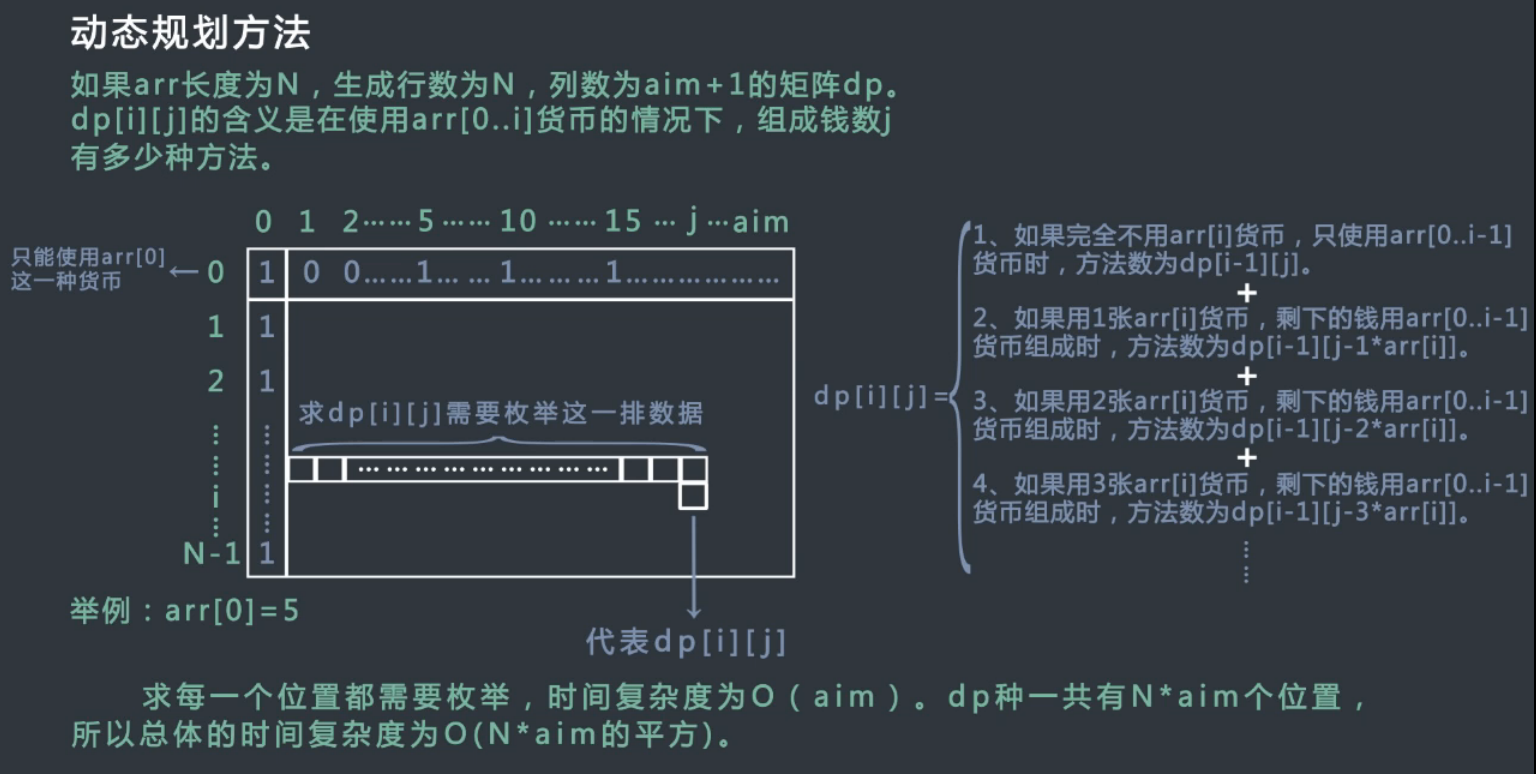从上图dp[i][j]的求解过程来看，它需要枚举上一行的数据，遍历dp[i-1][j-k*arr[i]，其中0<=k*arr[i]<=aim。 所需的时间复杂度为O(N∗aim2)<!--//--><![CDATA[//><!--
O(N*aim^2)
//--><!]]>。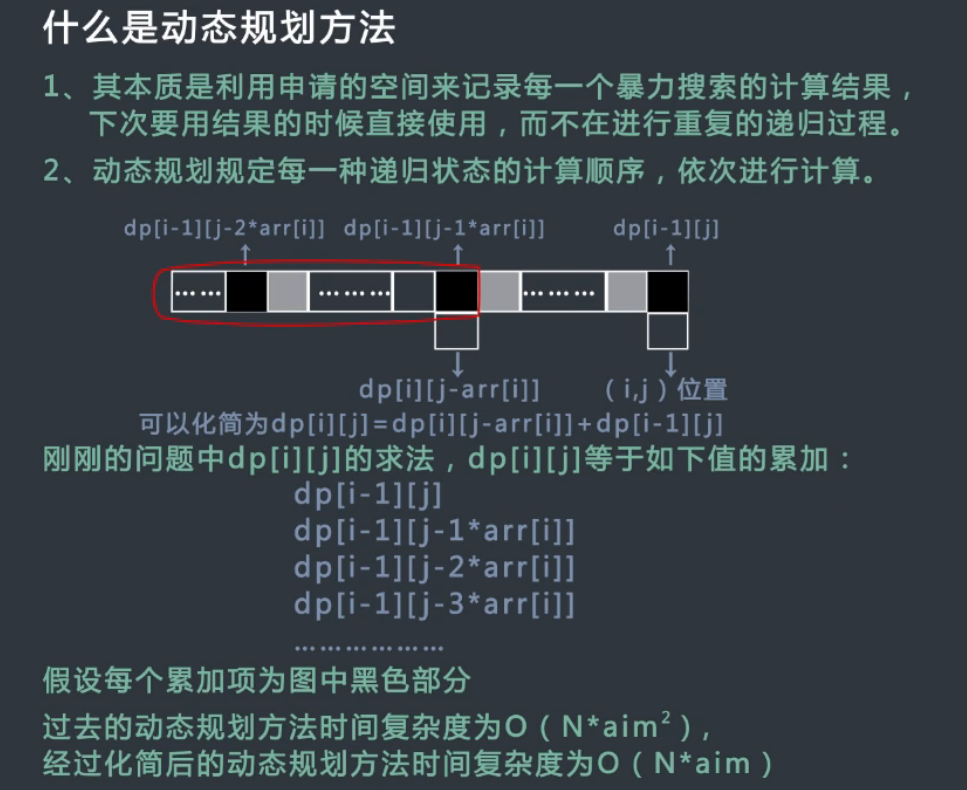显然，这样太过复杂，看下图，会发现上面的枚举的式子可以进一步改写为dp[i][j] = dp[i][j-arr[i]] + dp[i-1][j]，这样，之前的动态规划的复杂度经过化简后可以达到O(N∗aim)<!--//--><![CDATA[//><!--
O(N*aim)
//--><!]]>。

public int coins3(int[] arr, int aim) {
int[][] dp = new int[n][aim+1];
for(int i = 0; i < n; i++) {
dp[i] = 1;
}
for(int j = 0; j < aim+1; j++) {
if(j % penny == 0)
dp[j] = 1;
}
for(int i = 1; i < n; i++) {
for(int j = 1; j < aim+1; j++) {
if(j - penny[i] >= 0)
dp[i][j] = dp[i-1][j] + dp[i][j-penny[i]];
else
dp[i][j] = dp[i-1][j];
}
}
return dp[n-1][aim];
}

如上，是在时间复杂度上进行了优化，个状态之间的关系就非常简洁明了了。但是，我们还可以在空间复杂度上进行进一步优化，将二维状态矩阵使用一维矩阵替代。可以看到，动态规划在对求解顺序上有着非常严格的要求，上述状态求解就是从矩阵左上角依次从上到下，从左到右求解的过程。后面的状态依赖于前端的状态。因此，如果我们不需要存储中间的结果，只需要得到最终结果，那么完全可以舍弃对每一行状态的存储。但是，仍然需要严格遵守求解顺序。程序可以优化如下：

public int coins4(int[] penny, int n, int aim) {
int[] dp = new int[aim+1];
dp = 1;
for(int i = 0; i < n; i++) {
for(int j = penny[i]; j < aim+1; j++) {
dp[j] += dp[j - penny[i]];
}
}
return dp[aim];
}

程序的空间复杂度由O(n∗aim)<!--//--><![CDATA[//><!--
O(n*aim)
//--><!]]>简化到了O(aim)<!--//--><![CDATA[//><!--
O(aim)
//--><!]]>。

例题

题目全部来自于牛客网课程，代码为自己编写。

台阶问题

有n级台阶，一个人每次上一级或者两级，问有多少种走完n级台阶的方法。为了防止溢出，请将结果Mod 1000000007。

测试样例：
1
返回：1

public int countWays(int n) {
// write code here
int[] dp = new int[n+1];
dp = 0;
dp = 1;
dp = 2;
for(int i = 3; i < n+1; i++) {
dp[i] = (dp[i-1] + dp[i-2])%1000000007;
}
return dp[n];
}

矩阵最小路径和

有一个矩阵map，它每个格子有一个权值。从左上角的格子开始每次只能向右或者向下走，最后到达右下角的位置，路径上所有的数字累加起来就是路径和，返回所有的路径中最小的路径和。

给定一个矩阵map及它的行数n和列数m，请返回最小路径和。保证行列数均小于等于100.

测试样例：
[[1,2,3],[1,1,1]],2,3
返回：4

public int getMin(int[][] map, int n, int m) {
// write code here
int[][] dp = new int[n][m];
dp = map;
for(int i = 1; i < n; i++)
dp[i] += dp[i-1] + map[i];
for(int j = 1; j < m; j++)
dp[j] = dp[j-1] + map[j];
for(int i = 1; i < n; i++) {
for(int j = 1; j < m; j++) {
dp[i][j] = Math.min(dp[i][j-1], dp[i-1][j]) + map[i][j];
}
}
return dp[n-1][m-1];
}

LIS（最长上升子序列）

这是一个经典的LIS(即最长上升子序列)问题，请设计一个尽量优的解法求出序列的最长上升子序列的长度。

给定一个序列A及它的长度n(长度小于等于500)，请返回LIS的长度。

测试样例：
[1,4,2,5,3],5
返回：3

public int getLIS(int[] A, int n) {
// write code here
int[] dp = new int[n];
int max = 0;
for(int i = 0; i < n; i++) {
dp[i] = 1;
}
for(int i = 1; i < n; i++) {
for(int j = 0; j < i; j++) {
if(A[i] > A[j] && dp[i] < dp[j]+1)
dp[i] = dp[j] + 1;
}
}
for(int i = 0; i < n; i++) {
max = dp[i] > max ? dp[i] : max;
}
return max;
}

LCS（最长公共子序列）

给定两个字符串A和B，返回两个字符串的最长公共子序列的长度。例如，A=”1A2C3D4B56”，B=”B1D23CA45B6A”，”123456”或者”12C4B6”都是最长公共子序列。

给定两个字符串A和B，同时给定两个串的长度n和m，请返回最长公共子序列的长度。保证两串长度均小于等于300。

测试样例：
“1A2C3D4B56”,10,”B1D23CA45B6A”,12
返回：6

char[] a = A.toCharArray();
char[] b = B.toCharArray();
int[][] dp = new int[n][m];
boolean finda = false, findb = false;
for(int i = 0; i < n; i++) {
if(a[i] == b)
finda = true;
if(finda)
dp[i] = 1;
}
for(int i = 0; i < m; i++) {
if(b[i] == a)
findb = true;
if(findb)
dp[i] = 1;
}
for(int i = 1; i < n; i++) {
for(int j = 1; j < m; j++) {
if(a[i] == b[j]) {
dp[i][j] = dp[i-1][j-1] + 1;
} else {
dp[i][j] = Math.max(dp[i-1][j], dp[i][j-1]);
}
}
}
return dp[n-1][m-1];

01背包问题

一个背包有一定的承重cap，有N件物品，每件都有自己的价值，记录在数组v中，也都有自己的重量，记录在数组w中，每件物品只能选择要装入背包还是不装入背包，要求在不超过背包承重的前提下，选出物品的总价值最大。

给定物品的重量w价值v及物品数n和承重cap。请返回最大总价值。

测试样例：
[1,2,3],[1,2,3],3,6
返回：6

public int maxValue(int[] w, int[] v, int n, int cap) {
int[] dp = new int[cap+1];
for(int i = 0; i < n; i++) {
for(int j = cap; j >=w[i]; j--) {
dp[j] = Math.max(dp[j-w[i]] + v[i], dp[j]);
}
}
return dp[cap];
}

最优编辑问题

对于两个字符串A和B，我们需要进行插入、删除和修改操作将A串变为B串，定义c0，c1，c2分别为三种操作的代价，请设计一个高效算法，求出将A串变为B串所需要的最少代价。

给定两个字符串A和B，及它们的长度和三种操作代价，请返回将A串变为B串所需要的最小代价。保证两串长度均小于等于300，且三种代价值均小于等于100。

测试样例：
返回：8

public int findMinCost(String A, int n, String B, int m, int c0, int c1, int c2) {
// write code here
int[][] dp = new int[n][m];
char[] a = A.toCharArray();
char[] b = B.toCharArray();
boolean findb = false, finda = false;
for(int i = 0; i < n; i++) {
if(a[i] == b)
findb = true;
if(findb)
dp[i] = c1*i;
else
dp[i] = Math.min(c1*(i+1)+c0, c1*i+c2);
}
for(int j = 0; j < m; j++) {
if(a == b[j])
finda = true;
if(finda)
dp[j] = c0*j;
else
dp[j] = Math.min(c0*(j+1)+c1, c0*j+c2);
}
for(int i = 1; i < n; i++) {
for(int j = 1; j < m; j++) {
if(a[i] == b[j])
dp[i][j] = dp[i-1][j-1];
else
dp[i][j] = Math.min(Math.min(dp[i-1][j]+c1, dp[i][j-1]+c0),
Math.min(dp[i-1][j-1]+c2, dp[i-1][j-1]+c0+c1));
}
}
return dp[n-1][m-1];
}
展开全文• ## Java 动态规划

万次阅读 多人点赞 2019-06-23 16:16:43
Java中的动态规划 介绍 动态规划典型的被用于优化递归算法，因为它们倾向于以指数的方式进行扩展。动态规划主要思想是将复杂问题（带有许多递归调用）分解为更小的子问题，然后将它们保存到内存中，这样我们就不必在...
Java中的动态规划
介绍
动态规划典型的被用于优化递归算法，因为它们倾向于以指数的方式进行扩展。动态规划主要思想是将复杂问题（带有许多递归调用）分解为更小的子问题，然后将它们保存到内存中，这样我们就不必在每次使用它们时重新计算它们。
要理解动态规划的概念，我们需要熟悉一些主题：

什么是动态规划？
贪心算法
简化的背包问题
传统的背包问题
LCS-最长的共同子序列
利用动态规划的其他问题
结论

什么是动态规划？
动态规划是一种编程原理，可以通过将非常复杂的问题划分为更小的子问题来解决。这个原则与递归很类似，但是与递归有一个关键点的不同，就是每个不同的子问题只能被解决一次。
为了理解动态规划，我们首先需要理解递归关系的问题。每个单独的复杂问题可以被划分为很小的子问题，这表示我们可以在这些问题之间构造一个递归关系。
让我们来看一个我们所熟悉的例子：斐波拉契数列，斐波拉契数列的定义具有以下的递归关系：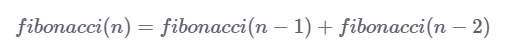注意：递归关系是递归地定义下一项是先前项的函数的序列的等式。Fibonacci序列就是一个很好的例子。
所以，如果我们想要找到斐波拉契数列序列中的第n个数，我们必须知道序列中第n个前面的两个数字。
但是，每次我们想要计算Fibonacci序列的不同元素时，我们在递归调用中都有一些重复调用，如下图所示，我们计算Fibonacci（5）：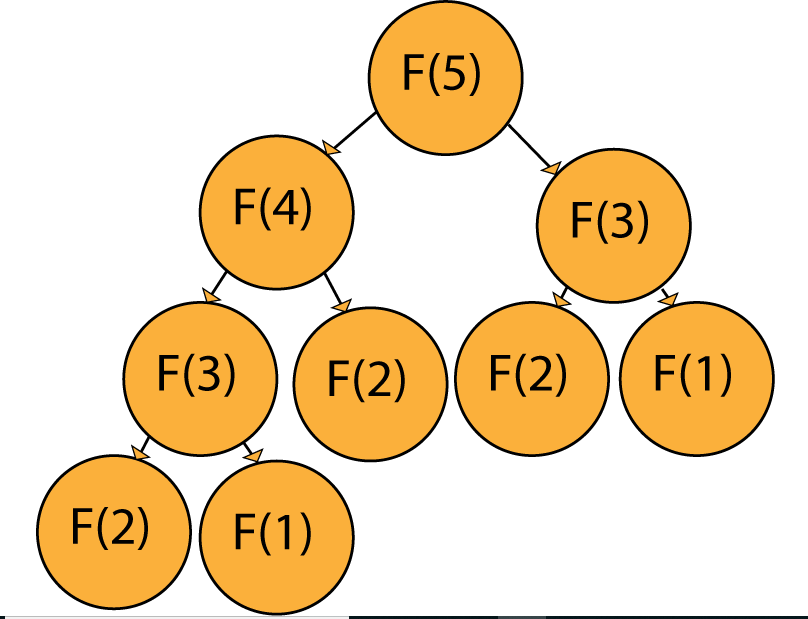例如：如果我们想计算F(5),明显的我们需要计算F(3)和F(4)作为计算F(5)的先决条件。然而，为了计算F(4)，我们需要计算F(3)和F(2)，因此我们又需要计算F(2)和F(1)来得到F(3)，其他的求解诸如此类。
这样的话就会导致很多重复的计算，这些重复计算本质上是冗余的，并且明显的减慢了算法的效率。为了解决这种问题，我们介绍动态规划。
在这种方法中，我们对解决方案进行建模，就像我们要递归地解决它一样，但我们从头开始解决它，将解决方案记忆到我们到达顶部的子问题（步骤）。
因此，对于Fibonacci序列，我们首先求解并记忆F（1）和F（2），然后使用两个记忆步骤计算F（3），依此类推。这意味着序列中每个单独元素的计算都是O（1），因为我们已经知道前两个元素。
当使用动态规划解决问题的时候，我们一般会采用下面三个步骤：

确定适用于所述问题的递归关系
初始化内存、数组、矩阵的初始值
确保当我们进行递归调用（可以访问子问题的答案）的时候它总是被提前解决。

遵循这些规则，让我们来看一下使用动态规划的算法的例子：
贪心算法
下面来以这个为例子：
Given a rod of length n and an array that contains prices of all pieces of size smaller than n. Determine the maximum value obtainable by cutting up the rod and selling the pieces.

###对于没有经验的开发者可能会采取下面这种做法：###
这个问题实际上是为动态规划量身定做的，但是因为这是我们的第一个真实例子，让我们看看通过运行这些代码运行来遇到多少问题：
public class naiveSolution {
static int getValue(int[] values, int length) {
if (length <= 0)
return 0;
int tmpMax = -1;
for (int i = 0; i < length; i++) {
tmpMax = Math.max(tmpMax, values[i] + getValue(values, length - i - 1));
}
return tmpMax;
}

public static void main(String[] args) {
int[] values = new int[]{3, 7, 1, 3, 9};
int rodLength = values.length;

System.out.println("Max rod value: " + getValue(values, rodLength));
}
}

输出结果：
Max rod value: 17
虽然这段代码得到的结果是正确的，按时代码的效率和很低。因为递归调用的结果不可以被保存因此代码不得不每次都去解决相同的子问题，这里就会存在一个重叠的答案。
动态方法
利用上面相同的基本原理，但添加memoization并排除递归调用，我们得到以下实现:
public class dpSolution {
static int getValue(int[] values, int rodLength) {
int[] subSolutions = new int[rodLength + 1];

for (int i = 1; i <= rodLength; i++) {
int tmpMax = -1;
for (int j = 0; j < i; j++)
tmpMax = Math.max(tmpMax, values[j] + subSolutions[i - j - 1]);
subSolutions[i] = tmpMax;
}
return subSolutions[rodLength];
}

public static void main(String[] args) {
int[] values = new int[]{3, 7, 1, 3, 9};
int rodLength = values.length;

System.out.println("Max rod value: " + getValue(values, rodLength));
}
}

输出结果：
Max rod value: 17
正如我们所看到的的，输出结果是一样的，所不同的是时间和空间复杂度。
通过从头开始解决子问题，我们消除了递归调用的需要，利用已解决给定问题的所有先前子问题的事实。
性能的提升
为了给出动态方法效率更高的观点的证据，让我们尝试使用30个值来运行该算法。 一种算法需要大约5.2秒来执行，而动态解决方法需要大约0.000095秒来执行。
简化的背包问题
简化的背包问题是一个优化问题，没有一个解决方案。这个问题的问题是 - “解决方案是否存在？”：
Given a set of items, each with a weight w1, w2... determine the number of each item to put in a knapsack so that the total weight is less than or equal to a given limit K.
首先让我们把元素的所有权重存储在W数组中。接下来，假设有n个项目，我们将使用从1到n的数字枚举它们，因此第i个项目的权重为W [i]。我们将形成（n + 1）x（K + 1）维的矩阵M。M [x] [y]对应于背包问题的解决方案，但仅包括起始数组的前x个项，并且最大容量为y
例如
假设我们有3个元素，权重分别是w1=2kg,w2=3kg,w3=4kg。利用上面的方法，我们可以说M  是一个有效的解决方案。
这意味着我们正在尝试用重量阵列中的第一个项目（w1）填充容量为2kg的背包。
在M  中，我们尝试使用重量阵列的前3项（w1，w2，w3）填充容量为5kg的背包。
这不是一个有效的解决方案，因为我们过度拟合它。
矩阵初始化
当初始化矩阵的时候有两点需要注意：
Does a solution exist for the given subproblem (M[x][y].exists) AND does the given solution include the latest item added to the array (M[x][y].includes).
因此，初始化矩阵是相当容易的，M[k].exists总是false，如果k>0，因为我们没有把任何物品放在带有k容量的背包里。
另一方面，M.exists = true,当k=0的时候，背包应该是空的，因此我们在里面没有放任何东西，这个是一个有效的解决方案。
此外，我们可以说M[k].exists = true，但是对于每个k来说    M[k].includes = false。
注意：仅仅因为对于给定的M [x] [y]存在解决方案，它并不一定意味着该特定组合是解决方案。
在M  的情况下，存在一种解决方案 - 不包括10个元素中的任何一个。
这就是M   .exists = true但M   .includes = false的原因。
算法原则
接下来，让我们使用以下伪代码构造M [i] [k]的递归关系：
if (M[i-1][k].exists == True):
M[i][k].exists = True
M[i][k].includes = False
elif (k-W[i]>=0):
if(M[i-1][k-W[i]].exists == true):
M[i][k].exists = True
M[i][k].includes = True
else:
M[i][k].exists = False

因此，解决方案的要点是将子问题分为两种情况:

对于容量k,当存在第一个i-1元素的解决方案
对于容量k-W [i],当第一个i-1元素存在解决方案

第一种情况是不言自明的，我们已经有了问题的解决方案。
实现：
下面这何种实现方式，使得事情变得更加容易，我们创建了一个类Element来存储元素：
public class Element {
private boolean exists;
private boolean includes;

public Element(boolean exists, boolean includes) {
this.exists = exists;
this.includes = includes;
}

public Element(boolean exists) {
this.exists = exists;
this.includes = false;
}

public boolean isExists() {
return exists;
}

public void setExists(boolean exists) {
this.exists = exists;
}

public boolean isIncludes() {
return includes;
}

public void setIncludes(boolean includes) {
this.includes = includes;
}
}

接着，我们可以深入了解主要的类：
public class Knapsack {
public static void main(String[] args) {
Scanner scanner = new Scanner (System.in);

System.out.println("Insert knapsack capacity:");
int k = scanner.nextInt();

System.out.println("Insert number of items:");
int n = scanner.nextInt();

System.out.println("Insert weights: ");
int[] weights = new int[n + 1];

for (int i = 1; i <= n; i++) {
weights[i] = scanner.nextInt();
}

Element[][] elementMatrix = new Element[n + 1][k + 1];

elementMatrix = new Element(true);

for (int i = 1; i <= k; i++) {
elementMatrix[i] = new Element(false);
}

for (int i = 1; i <= n; i++) {
for (int j = 0; j <= k; j++) {
elementMatrix[i][j] = new Element(false);
if (elementMatrix[i - 1][j].isExists()) {
elementMatrix[i][j].setExists(true);
elementMatrix[i][j].setIncludes(false);
} else if (j >= weights[i]) {
if (elementMatrix[i - 1][j - weights[i]].isExists()) {
elementMatrix[i][j].setExists(true);
elementMatrix[i][j].setIncludes(true);
}
}
}
}

System.out.println(elementMatrix[n][k].isExists());
}
}

唯一剩下的就是解决方案的重建，在上面的类中，我们知道解决方案是存在的，但是我们不知道它是什么。
为了重建，我们使用下面的代码：
List<Integer> solution = new ArrayList<>(n);

if (elementMatrix[n][k].isExists()) {
int i = n;
int j = k;
while (j > 0 && i > 0) {
if (elementMatrix[i][j].isIncludes()) {
j = j - weights[i];
}
i = i - 1;
}
}

System.out.println("The elements with the following indexes are in the solution:\n" + (solution.toString()));

输出：
Insert knapsack capacity:
12
Insert number of items:
5
Insert weights:
9 7 4 10 3
true
The elements with the following indexes are in the solution:
[5, 1]

背包问题的一个简单变化是在没有价值优化的情况下填充背包，但现在每个单独项目的数量无限。
通过对现有代码进行简单调整，可以解决这种变化：
// Old code for simplified knapsack problem
else if (j >= weights[i]) {
if (elementMatrix[i - 1][j - weights[i]].isExists()) {
elementMatrix[i][j].setExists(true);
elementMatrix[i][j].setIncludes(true);
}
}

// New code, note that we're searching for a solution in the same
// row (i-th row), which means we're looking for a solution that
// already has some number of i-th elements (including 0) in it's solution
else if (j >= weights[i]) {
if (elementMatrix[i][j - weights[i]].isExists()) {
elementMatrix[i][j].setExists(true);
elementMatrix[i][j].setIncludes(true);
}
}

传统的背包问题
利用以前的两种变体，现在让我们来看看传统的背包问题，看看它与简化版本的不同之处：
Given a set of items, each with a weight w1, w2... and a value v1, v2... determine the number of each item to include in a collection so that the total weight is less than or equal to a given limit k and the total value is as large as possible.

在简化版中，每个解决方案都同样出色。但是，现在我们有一个找到最佳解决方案的标准（也就是可能的最大值）。请记住，这次我们每个项目都有无限数量，因此项目可以在解决方案中多次出现。
在实现中，我们将使用旧的类Element，其中添加了私有字段value，用于存储给定子问题的最大可能值:
public class Element {
private boolean exists;
private boolean includes;
private int value;
// appropriate constructors, getters and setters
}

实现非常相似，唯一的区别是现在我们必须根据结果值选择最佳解决方案：
public static void main(String[] args) {
// Same code as before with the addition of the values[] array
System.out.println("Insert values: ");
int[] values = new int[n + 1];

for (int i=1; i <= n; i++) {
values[i] = scanner.nextInt();
}

Element[][] elementMatrix = new Element[n + 1][k + 1];

// A matrix that indicates how many newest objects are used
// in the optimal solution.
// Example: contains indicates how many objects with
// the weight of W are contained in the optimal solution
// for a knapsack of capacity K=10
int[][] contains = new int[n + 1][k + 1];

elementMatrix = new Element(0);

for (int i = 1; i <= n; i++) {
elementMatrix[i] = new Element(0);
contains[i] = 0;
}

for (int i = 1; i <= k; i++) {
elementMatrix[i] = new Element(0);
contains[i] = 0;
}

for (int i = 1; i <= n; i++) {
for (int j = 0; j <= k; j++) {
elementMatrix[i][j] = new Element(elementMatrix[i - 1][j].getValue());
contains[i][j] = 0;

elementMatrix[i][j].setIncludes(false);
elementMatrix[i][j].setValue(M[i - 1][j].getValue());

if (j >= weights[i]) {
if ((elementMatrix[i][j - weights[i]].getValue() > 0 || j == weights[i])) {
if (elementMatrix[i][j - weights[i]].getValue() + values[i] > M[i][j].getValue()) {
elementMatrix[i][j].setIncludes(true);
elementMatrix[i][j].setValue(M[i][j - weights[i]].getValue() + values[i]);
contains[i][j] = contains[i][j - weights[i]] + 1;
}
}
}

System.out.print(elementMatrix[i][j].getValue() + "/" + contains[i][j] + "  ");
}

System.out.println();
}

System.out.println("Value: " + elementMatrix[n][k].getValue());
}

输出：
Insert knapsack capacity:
12
Insert number of items:
5
Insert weights:
9 7 4 10 3
Insert values:
1 2 3 4 5
0/0  0/0  0/0  0/0  0/0  0/0  0/0  0/0  0/0  1/1  0/0  0/0  0/0
0/0  0/0  0/0  0/0  0/0  0/0  0/0  2/1  0/0  1/0  0/0  0/0  0/0
0/0  0/0  0/0  0/0  3/1  0/0  0/0  2/0  6/2  1/0  0/0  5/1  9/3
0/0  0/0  0/0  0/0  3/0  0/0  0/0  2/0  6/0  1/0  4/1  5/0  9/0
0/0  0/0  0/0  5/1  3/0  0/0  10/2  8/1  6/0  15/3  13/2  11/1  20/4
Value: 20

Levenshtein Distance
另一个使用动态规划的非常好的例子是Edit Distance或Levenshtein Distance。
Levenshtein Distance就是两个字符串A,B,我们需要使用原子操作将A转换为B：

字符串删除
字符串插入
字符替换（从技术上讲，它不止一个操作，但为了简单起见，我们称之为原子操作）

这个问题是通过有条理地解决起始字符串的子串的问题来处理的，逐渐增加子字符串的大小，直到它们等于起始字符串。
我们用于此问题的递归关系如下：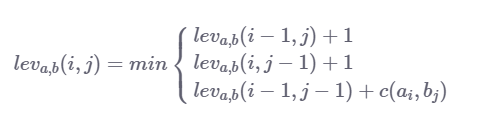如果a == b则c（a，b）为0，如果a = = b则c（a，b）为1。
实现：
public class editDistance {
public static void main(String[] args) {
String s1, s2;
Scanner scanner = new Scanner(System.in);
System.out.println("Insert first string:");
s1 = scanner.next();
System.out.println("Insert second string:");
s2 = scanner.next();

int n, m;
n = s1.length();
m = s2.length();

// Matrix of substring edit distances
// example: distance[a][b] is the edit distance
// of the first a letters of s1 and b letters of s2
int[][] distance = new int[n + 1][m + 1];

// Matrix initialization:
// If we want to turn any string into an empty string
// the fastest way no doubt is to just delete
// every letter individually.
// The same principle applies if we have to turn an empty string
// into a non empty string, we just add appropriate letters
// until the strings are equal.
for (int i = 0; i <= n; i++) {
distance[i] = i;
}
for (int j = 0; j <= n; j++) {
distance[j] = j;
}

// Variables for storing potential values of current edit distance
int e1, e2, e3, min;

for (int i = 1; i <= n; i++) {
for (int j = 1; j <= m; j++) {
e1 = distance[i - 1][j] + 1;
e2 = distance[i][j - 1] + 1;
if (s1.charAt(i - 1) == s2.charAt(j - 1)) {
e3 = distance[i - 1][j - 1];
} else {
e3 = distance[i - 1][j - 1] + 1;
}
min = Math.min(e1, e2);
min = Math.min(min, e3);
distance[i][j] = min;
}

}

System.out.println("Edit distance of s1 and s2 is: " + distance[n][m]);
}
}

输出：
Insert first string:
man
Insert second string:
machine
Edit distance of s1 and s2 is: 3

最长共同子序列（LCS）
这个问题描述如下：
Given two sequences, find the length of the longest subsequence present in both of them. A subsequence is a sequence that appears in the same relative order, but not necessarily contiguous.

给定两个序列，找到两个序列中存在的最长子序列的长度。子序列是以相同的相对顺序出现的序列，但不一定是连续的.
阐明：
如果我们有两个字符串s1="MICE"和s2="MINCE",最长的共同子序列是MI或者CE。但是，最长的公共子序列将是“MICE”，因为结果子序列的元素不必是连续的顺序。
递归关系与一般逻辑：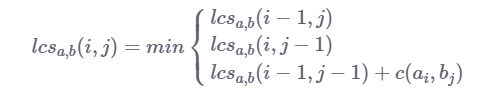我们可以看到，Levenshtein distance和LCS之间只有微小的差别，特别是移动成本。
在LCS中，我们没有字符插入和字符删除的成本，这意味着我们只计算字符替换（对角线移动）的成本，如果两个当前字符串字符a [i]和b [j] 是相同的,则成本为1。
LCS的最终成本是2个字符串的最长子序列的长度，这正是我们所需要的。
Using this logic, we can boil down a lot of string comparison algorithms to simple recurrence relations which utilize the base formula of the Levenshtein distance
使用这个逻辑，我们可以将许多字符串比较算法归结为简单的递归关系，它使用Levenshtein distance的基本公式。
实现：
public class LCS {
public static void main(String[] args) {
String s1 = new String("Hillfinger");
String s2 = new String("Hilfiger");
int n = s1.length();
int m = s2.length();
int[][] solutionMatrix = new int[n+1][m+1];
for (int i = 0; i < n; i++) {
solutionMatrix[i] = 0;
}
for (int i = 0; i < m; i++) {
solutionMatrix[i] = 0;
}

for (int i = 1; i <= n; i++) {
for (int j = 1; j <= m; j++) {
int max1, max2, max3;
max1 = solutionMatrix[i - 1][j];
max2 = solutionMatrix[i][j - 1];
if (s1.charAt(i - 1) == s2.charAt(j - 1)) {
max3 = solutionMatrix[i - 1][j - 1] + 1;
} else {
max3 = solutionMatrix[i - 1][j - 1];
}
int tmp = Math.max(max1, max2);
solutionMatrix[i][j] = Math.max(tmp, max3);
}
}

System.out.println("Length of longest continuous subsequence: " + solutionMatrix[n][m]);
}
}

输出：
Length of longest continuous subsequence: 8

利用动态规划的其他问题:
利用动态规划可以解决很多问题，下面列举了一些：

分区问题：给定一组整数，找出它是否可以分成两个具有相等和的子集
子集和问题：给你一个正整数的数组及元素还有一个合计值，是否在数组中存在一个子集的的元素之和等于合计值。
硬币变化问题：鉴于给定面额的硬币无限供应，找到获得所需变化的不同方式的总数
k变量线性方程的所有可能的解：给定k个变量的线性方程，计算它的可能解的总数
找到醉汉不会从悬崖上掉下来的概率：给定一个线性空间代表距离悬崖的距离，让你知道酒鬼从悬崖起始的距离，以及他向悬崖p前进并远离悬崖1-p的倾向，计算出他的生存概率

##结论：##
动态编程是一种工具，可以节省大量的计算时间，以换取更大的空间复杂性，这在很大程度上取决于您正在处理的系统类型，如果CPU时间很宝贵，您选择耗费内存的解决方案，另一方面，如果您的内存有限，则选择更耗时的解决方案
原文：https://stackabuse.com/dynamic-programming-in-java/
译者：lee展开全文背包问题
• ## 动态规划拙见

千次阅读 2021-01-24 00:01:20
动态规划 刷题也有好几个月了，但一直都是沉醉于数组和字符串这些入门的知识点，这假期准备好好啃一些知名的算法了 动态规划作为科技公司基本上必考的知识点，在刷题网站中占有很大的比重，很多的题目都可以用动态...leetcode python
• 对于动态规划，春招秋招时好多题都会用到动态规划，一气之下，再 leetcode 连续刷了几十道动态规划的题在这里插入图片描述之后，豁然开朗 ，感觉动态规划也不是很难，今天...
• ## 动态规划快速入门

千次阅读 2019-05-19 16:07:56
动态规划算法一直是面试手撕算法中比较有挑战的一种类型。很多的分配问题或者调度问题实际上都可能用动态规划进行解决。（当然，如果问题的规模较大，有时候会抽象模型使用动归来解决，有时候则可以通过不断迭代的...java
• 动态规划（Dynamicprogramming） 是一种在数学、计算机科学和经济学中使用的，通过把原问题分解为相对简单的子问题的方式求解复杂问题的方法。动态规划算法是通过拆分问题，定义问题状态和状态之间的关系，使得问题...
• 动态规划与其他的算法不一样，动态规划是没有一个固定的模板的，它只是一种思想，并不是一种特殊算法。 那么，动态规划既然没有一个固定的模板，那怎么来分析它呢？或者说，做出来了怎么写题解呢？这里给大家介绍一...dp
• ## 动态规划常见类型总结

千次阅读 多人点赞 2019-03-26 23:55:28
严格来说，递推不属于动态规划问题，因为动态规划不仅有递推过程，还要有决策（即取最优），但广义的动态规划是可以包含递推的，递推是一类简单的、特殊的动态规划，毕竟动态规划与递推密不可分。动态规划类型主要...DP NOIP NOI
• 虽然我们已经用动态规划方法解决了钢条切割和矩阵链乘法两个问题，但你可能还是弄不清应该在何时使用动态规划。我们关注适合应用动态规划方法求解的最优化问题应该具备的两个要素：最优子结构和子问题重叠。我们还会...算法 算法分析
• 动态规划程序设计是对解最优化问题的一种途径、一种方法，而不是一种特殊算法。算法入门 算法详解
• 作者：王勐 ...来源：知乎 著作权归作者所有。商业转载请联系作者获得授权，非商业转载请注明出处。...理解动态规划并不需要数学公式介入，只是完全解释清楚需要点篇幅…首先需要明白哪些问题不是动态规划可以解决
• ## 动态规划DP

千次阅读 2016-12-03 09:40:18
一.算法思想 和贪婪算法一样，在动态规划中，可将一个...不同的是，在贪婪算法中，每采用一次贪婪准则便做出一个不可撤回的决策，而在动态规划中，还要考察每个最优决策序列中是否包含一个最优子序列。 动态规划
• 动态规划算法与分治法类似，其基本思想也是将待求解问题分解成若干个子问题，先求解子问题，然后从这些子问题的解得到原问题的解。与分治法不同的是，适合于用动态规划求解的问题，经分解得到子问题往往不是互相独立...最长公共子序列
• ## 动态规划算法

千次阅读 2018-03-03 16:53:41
动态规划算法关键字：子结构 状态转移方程 数塔 背包问题 目录 一、前言二、动态规划概述 1. 定义 2. 概念 3. 求解基本步骤 4. 谈谈动态规划与其他算法之间的联系和区别 5. 动态规划迷思 6. 动态规划...
• ## 动态规划算法介绍

千次阅读 2019-02-17 19:34:49
动态规划算法及其应用 动态规划是运筹学的一个分支，是求解决策过程最优化的数学方法。动态规划算法的思想和分治算法类似，基本思想也是将待求问题分解成若干个子问题，先求解子问题，然后从这些子问题的解得到原...
• ## 动态规划入门

千次阅读 2021-02-27 18:10:45
首先用最经典的一道题来引入动态规划 动态规划（dynamic programming） 请看下面这个题 一个十分漂亮的数字三角形；求哪条路径能使得各个数字的和最大； 如果我们只是单纯的贪心，即每次选择最大值走， 那么结果...算法
• ## 动态规划简介

千次阅读 2015-09-06 17:06:06
动态规划 动态规划 是一个多阶段决策过程： 关于动态规划的几个概念 决策：在一个阶段的状态给定以后，从该状态演变到下一阶段某个状态的一种选择称为决策。也就是在两个阶段间选择发展途径的行为。 决策变量：...
• ## 选课——动态规划

千次阅读 2017-09-25 15:12:33
• ## 动态规划模板总结

千次阅读 2018-08-06 17:06:47
动态规划： 通过把原问题分解为相对简单的子问题的方式求解复杂问题的方法。 动态规划常常适用于有重叠子问题和最优子结构性质的问题，动态规划方法所耗时间往往远少于朴素解法。 试用情况： 最优子结构性质。...
• 动态规划相信大家都知道，动态规划算法也是新手在刚接触算法设计时很苦恼的问题，有时候觉得难以理解，但是真正理解之后，就会觉得动态规划其实并没有想象中那么难。网上也有很多关于讲解动态规划的文章，大多都是...
• ## 区间动态规划详解

千次阅读 2018-11-15 20:57:40
前段时间遇到石子合并问题，看着题解A了，以为学会了区间DP，再次遇到能量项链这个问题的时候大脑还是一片空白，只能重新认识一下区间动态规划了。 翻过很多博客，基本上都是题解，真正对区间动态规划本身的讲解...区间DP 石子合并
• ## 动态规划题目

千次阅读 2018-05-29 16:00:07
109. 数字三角形 给定一个数字三角形，找到从顶部到底部的最小路径和。每一步可以移动到下面一行的相邻数字上。 样例 比如，给出下列数字三角形： ...最简单的动态规划思想： 从底层逐层向上计算最小路径并保存...